# Fraction calculator

The calculator performs basic and advanced operations with fractions, expressions with fractions combined with integers, decimals, and mixed numbers. It also shows detailed step-by-step information about the fraction calculation procedure. Solve problems with two, three, or more fractions and numbers in one expression.

## Result:

### 1/2 * 1/2 * 1/2 * 1/2 = 1/16 = 0.0625

Spelled result in words is one sixteenth.

### How do you solve fractions step by step?

1. Multiple: 1/2 * 1/2 = 1 · 1/2 · 2 = 1/4
Multiply both numerators and denominators. Result fraction keep to lowest possible denominator GCD(1, 4) = 1. In the following intermediate step, it cannot further simplify the fraction result by canceling.
In other words - one half multiplied by one half = one quarter.
2. Multiple: the result of step No. 1 * 1/2 = 1/4 * 1/2 = 1 · 1/4 · 2 = 1/8
Multiply both numerators and denominators. Result fraction keep to lowest possible denominator GCD(1, 8) = 1. In the following intermediate step, it cannot further simplify the fraction result by canceling.
In other words - one quarter multiplied by one half = one eighth.
3. Multiple: the result of step No. 2 * 1/2 = 1/8 * 1/2 = 1 · 1/8 · 2 = 1/16
Multiply both numerators and denominators. Result fraction keep to lowest possible denominator GCD(1, 16) = 1. In the following intermediate step, it cannot further simplify the fraction result by canceling.
In other words - one eighth multiplied by one half = one sixteenth.

#### Rules for expressions with fractions:

Fractions - simply use a forward slash between the numerator and denominator, i.e., for five-hundredths, enter 5/100. If you are using mixed numbers, be sure to leave a single space between the whole and fraction part.
The slash separates the numerator (number above a fraction line) and denominator (number below).

Mixed numerals (mixed fractions or mixed numbers) write as integer separated by one space and fraction i.e., 1 2/3 (having the same sign). An example of a negative mixed fraction: -5 1/2.
Because slash is both signs for fraction line and division, we recommended use colon (:) as the operator of division fractions i.e., 1/2 : 3.

Decimals (decimal numbers) enter with a decimal point . and they are automatically converted to fractions - i.e. 1.45.

The colon : and slash / is the symbol of division. Can be used to divide mixed numbers 1 2/3 : 4 3/8 or can be used for write complex fractions i.e. 1/2 : 1/3.
An asterisk * or × is the symbol for multiplication.
Plus + is addition, minus sign - is subtraction and ()[] is mathematical parentheses.
The exponentiation/power symbol is ^ - for example: (7/8-4/5)^2 = (7/8-4/5)2

#### Examples:

subtracting fractions: 2/3 - 1/2
multiplying fractions: 7/8 * 3/9
dividing Fractions: 1/2 : 3/4
exponentiation of fraction: 3/5^3
fractional exponents: 16 ^ 1/2
adding fractions and mixed numbers: 8/5 + 6 2/7
dividing integer and fraction: 5 ÷ 1/2
complex fractions: 5/8 : 2 2/3
decimal to fraction: 0.625
Fraction to Decimal: 1/4
Fraction to Percent: 1/8 %
comparing fractions: 1/4 2/3
multiplying a fraction by a whole number: 6 * 3/4
square root of a fraction: sqrt(1/16)
reducing or simplifying the fraction (simplification) - dividing the numerator and denominator of a fraction by the same non-zero number - equivalent fraction: 4/22
expression with brackets: 1/3 * (1/2 - 3 3/8)
compound fraction: 3/4 of 5/7
fractions multiple: 2/3 of 3/5
divide to find the quotient: 3/5 ÷ 2/3

The calculator follows well-known rules for order of operations. The most common mnemonics for remembering this order of operations are:
PEMDAS - Parentheses, Exponents, Multiplication, Division, Addition, Subtraction.
BEDMAS - Brackets, Exponents, Division, Multiplication, Addition, Subtraction
BODMAS - Brackets, Of or Order, Division, Multiplication, Addition, Subtraction.
GEMDAS - Grouping Symbols - brackets (){}, Exponents, Multiplication, Division, Addition, Subtraction.
Be careful, always do multiplication and division before addition and subtraction. Some operators (+ and -) and (* and /) has the same priority and then must evaluate from left to right.

## Fractions in word problems:

• Equation 20In given equation: 8/9-4/5=2/9+x, find x7 is added to the sum of 4/5 and 6/7
• Find N 2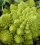Find N = 1 4/5 ÷ 3/5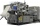Erika’s career consists of filing, typing and answering phones. She spends 1/6 of her time filing and 5/8 of her time typing. What fraction of her time does she spend answering phone calls?
• MichaelMichael had a bar of chocolate. He ate 1/2 of it and gave away 1/3. What fraction had he left?3 3/4 + 2 3/5 + 5 1/2 Show your solution.
• What is one third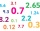What is 1/3 as a decimal? Give your answer rounded to 2 decimal places.
• A korean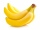A korean mart sells banana milk, strawberry milk, and melon milk. 65% of the milks sold is banana and 14% is strawberry. What percentage is melon milk?
• Tyreek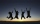Tyreek, Antuan and Alex are given a total of \$3500 to be shared among themselves in the ratio 3:5:12. How much each get money?
• Orchard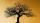3/5 of the trees in the orchard are apples, 1/3 are cherries and the remaining five trees are pear. How many trees are in the orchard?
• Holiday tripPavel is saving for the holiday trip. He received 1100 CZK from his father. He saved a fifth himself and received the rest 500 CZK from his mother. How much did his holiday trip cost?
• Calories 2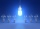Ben eats approximately 2400 calories per day. His wife Sarah eats 5/8 as much. How many calories does Sarah eat per day?
• Visit to grandfatherShane's family traveled 3/10 of the distance to his grandfather’s house on Saturday. They traveled 4/7 of the remaining distance on Sunday. What fraction of the total distance to his grandfather’s house was traveled on Sunday?